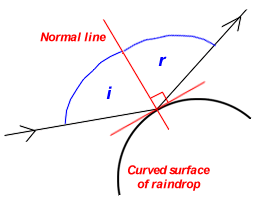Regular and diffuse reflection
Reflection is what allows us to see things. Light, from a souce such as a light globe or the Sun, strikes the objects and is reflected back to our eyes. There are two main types of reflection: diffuse reflection and regular reflection. See the diagram below.Copyright Rebecca McDowell 1999

The Law of Reflection
Regular reflection follows the Law of Reflection. The Law of Reflection states that the angle of incidence (i) and the angle of reflection (r) are equal. The angle of incidence is the angle between the incoming ray and the normal line. The angle of reflection is the angle between the reflected ray and the normal line.
Stated simply, i = r. This is demonstrated in the diagram below.Reflection and rainbows  This law is obeyed twice in the formation of a rainbow. The surface of a water droplet is smooth but curved. When it reflects light, it behaves like an infinite number of small, flat mirrors, each of which obeys the law of reflection. See right.  The law of reflection is also obeyed at the rear of the water drop when total internal reflection occurs.